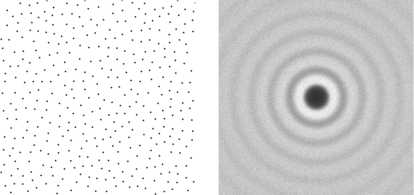CR类别： I.3.0 [计算机图形]：综合

#### 2. 算法1：从算法得出的二维样本模式及相关多行平均周期图谱

step 0. 初始化一个 n 维背景网格，用于储存样本，加速空间搜索。我们选择单元格尺寸受限于 r / $$\sqrt{n}$$，这样每个单元格都将最多包含一个样本，网格可以用一个简单的 n 维数组来实现：默认 -1 表示没有样本，一个非负整数表示一个单元格内样本的索引。

step 1. 从样本域里均匀随机选择初始样本 X0。将其插入背景网格，用该索引（0）初始化 “活动列表”（样本索引的数组）。

step 2. 当 活动列表 不为空时，从中选择一个随机索引（称为 i）。从距离 Xi 半径 r2r 之间的球形环中均匀地生成 k 个点。依次检查每个点是否位于现有样本的 r 距离内（背景网格只用于检测附近样本）。如果某个点距离现有样本足够远，将其作为下一个样本，并添加至活动列表中。如果尝试 k 次之后未发现这种点，则从活动列表中移除 i

#### 3. 分析

 123456789101112131415161718192021222324252627282930313233343536373839404142434445464748495051525354555657585960616263646566676869707172737475767778798081828384858687888990919293949596979899100101102103104105106107108109110111112113114115116117118119120121122123124125126127128129130131132133134135136137138139140 #ifndef BLUENOISE_H #define BLUENOISE_H #include #include // Provides type Vec, a C++ wrapper around T[N] to // encapsulate N-dimensional vectors. #include "vec.h" // Produce a sample uniformly chosen from the annulus between // (radius,2*radius] around center x. Uses random() for underlying PRNG. template void sample_annulus(T radius, const Vec ¢re, Vec &x) { Vec r; for(;;){ // simple rejection sampling approach for(unsigned int i=0; i1 && r2<=4) break; } x=centre+radius*r; } // Translate a multidimensional array index into a one dimensional array index. // (rounds towards zero if T is float or double) template unsigned long int n_dimensional_array_index(const Vec &dimensions, const Vec &x) { unsigned long int k=0; for(unsigned int i=N; i>0; --i){ k*=dimensions[i-1]; if(x[i-1]>=0){ unsigned int j=(int)x[i-1]; if(j>=dimensions[i-1]) j=dimensions[i-1]-1; k+=j; } } return k; } // Generate blue noise samples at least radius apart in N dimensions // within box bounded by xmin and xmax. T should either be float or double. template void blue_noise_sample(T radius, Vec xmin, Vec xmax, std::vector > &sample, // contains samples on return int max_sample_attempts=30) { // initialize acceleration grid (step 0) T grid_dx=T(0.99999)*radius/std::sqrt((T)N); Vec dimensions; unsigned long int total_array_size=1; for(unsigned int i=0; i accel(total_array_size, -1); // -1 indicates no sample there; // otherwise index of sample point // first sample (step 1) Vec x; for(unsigned int i=0; i active_list; active_list.push_back(0); unsigned int k=n_dimensional_array_index(dimensions, (x-xmin)/grid_dx); accel[k]=0; // generate the remaining samples (step 2) while(!active_list.empty()){ unsigned int r=(unsigned int)((random()/(T)2147483648u)*active_list.size()); int p=active_list[r]; bool found_sample=false; Vec j, jmin, jmax; for(int attempt=0; attemptxmax[i]) goto reject_sample; } // find range in acceleration grid that may contain interfering samples for(unsigned int i=0; i=(int)dimensions[i]) thismin=dimensions[i]-1; jmin[i]=(unsigned int)thismin; int thismax=(int)((x[i]+radius-xmin[i])/grid_dx); if(thismax<0) thismax=0; else if(thismax>=(int)dimensions[i]) thismax=dimensions[i]-1; jmax[i]=(unsigned int)thismax; } // loop over the selected grid cells (a little obfuscated since this is, // in effect, a nested N-dimensional loop) for(j=jmin;;){ // check if there's a sample at j that's too close to x k=n_dimensional_array_index(dimensions, j); if(accel[k]>=0 && accel[k]!=p){ // if there is a sample different from p if(dist2(x, sample[accel[k]]) < radius*radius) goto reject_sample; // proposed sample is too close to accel[k] } // move on to next j (N-dimensional nested version of ++j) for(unsigned int i=0; i

javascript 实现: https://github.com/kchapelier/poisson-disk-sampling